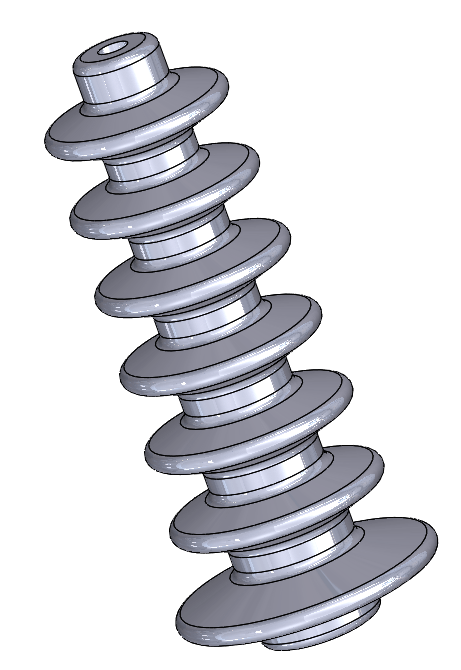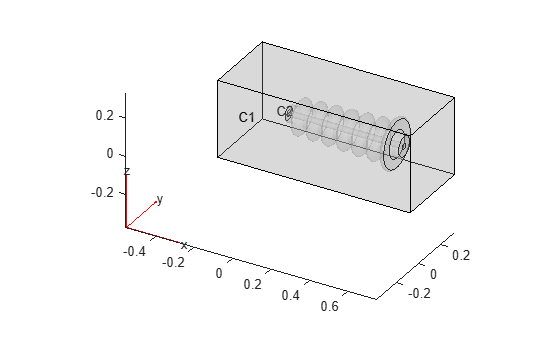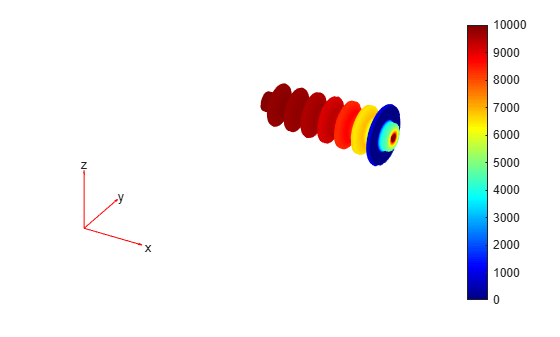Electrostatic Analysis of Transformer Bushing Insulator

This example shows how to compute the electric field intensity in a bushing insulator of a transformer. Bushing insulators must withstand large electric fields due to the potential difference between the ground and the high-voltage conductor. This example uses a 3-D electrostatic model to compute the voltage distribution and electric field intensity in the bushing.Create an electromagnetic model for electrostatic analysis.

model = createpde('electromagnetic','electrostatic');

Import and plot the bushing geometry.

gmBushing = importGeometry('TransformerBushing.stl');
pdegplot(gmBushing)Model the surrounding air as a cuboid, and position the cuboid to contain the bushing at its center.

gmAir = multicuboid(1,0.4,0.4);
gmAir.translate([0.25,0.125,-0.07]);

Plot the resulting geometry with the cell labels.

pdegplot(gmModel,'CellLabels','on','FaceAlpha',0.25)Include the geometry in the model.

model.Geometry = gmModel;

Specify the vacuum permittivity value in the SI system of units.

model.VacuumPermittivity = 8.8541878128E-12;

Specify the relative permittivity of the air.

electromagneticProperties(model,'Cell',1,'RelativePermittivity',1);

Specify the relative permittivity of the bushing insulator.

electromagneticProperties(model,'Cell',2,'RelativePermittivity',5);

Before specifying boundary conditions, identify the face IDs by plotting the geometry with the face labels. To see the IDs more clearly, rotate the geometry.

pdegplot(gmModel,'FaceLabels','on','FaceAlpha',0.2)
view([55 5])Specify the voltage boundary condition on the inner walls of the bushing exposed to conductor.

electromagneticBC(model,'Face',12,'Voltage',10E3);

Specify the grounding boundary condition on the surface in contact with the oil tank.

electromagneticBC(model,'Face',9,'Voltage',0);

Generate a mesh and solve the model.

generateMesh(model);
R = solve(model)
R =
ElectrostaticResults with properties:

ElectricPotential: [40956x1 double]
ElectricField: [1x1 FEStruct]
ElectricFluxDensity: [1x1 FEStruct]
Mesh: [1x1 FEMesh]

Plot the voltage distribution in the bushing.

elemsBushing = findElements(model.Mesh,'Region','Cell',2);
pdeplot3D(model.Mesh.Nodes, ...
model.Mesh.Elements(:,elemsBushing), ...
'ColorMapData',R.ElectricPotential);Plot the magnitude of the electric field intensity in the bushing.

Emag = sqrt(R.ElectricField.Ex.^2 + ...
R.ElectricField.Ey.^2 + ...
R.ElectricField.Ez.^2);
pdeplot3D(model.Mesh.Nodes, ...
model.Mesh.Elements(:,elemsBushing), ...
'ColorMapData',Emag);Partial Differential Equation Toolbox DocumentationGet trial now